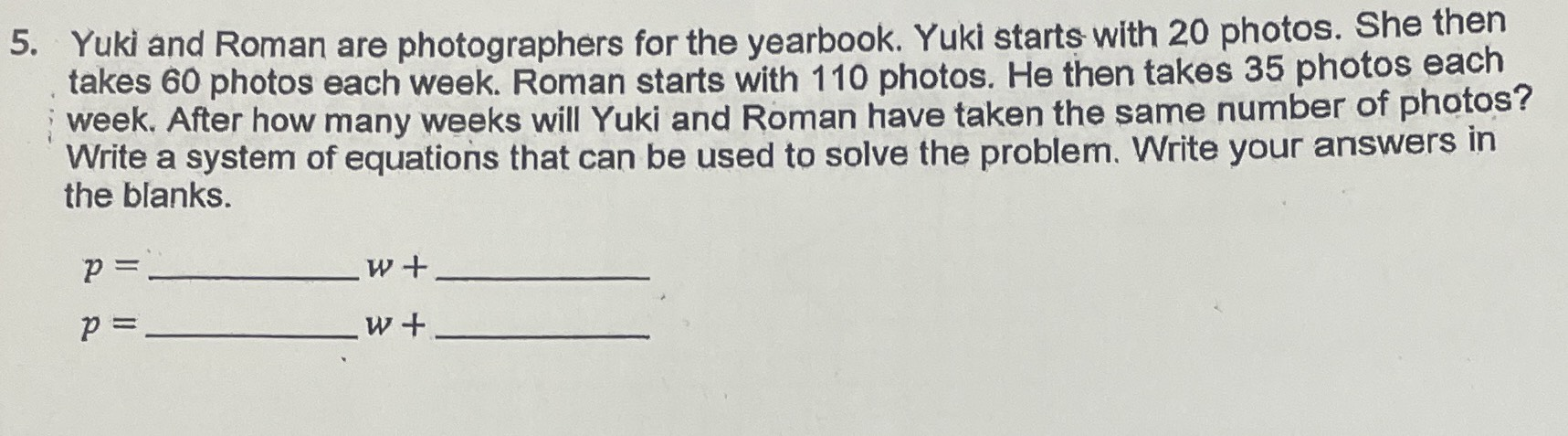### ¿Todavía tienes preguntas de matemáticas?

Pregunte a nuestros tutores expertos
Algebra
Pregunta5. Yuki and Roman are photographers for the yearbook. Yuki starts with $$20$$ photos. She then takes $$60$$ photos each week. Roman starts with $$110$$ photos. He then takes $$35$$ photos each week. After how many weeks will Yuki and Roman have taken the same number of photos? Write a system of equations that can be used to solve the problem. Write your answers in the blanks.

$$p =$$ ____w+____

$$p =$$ ____w+____